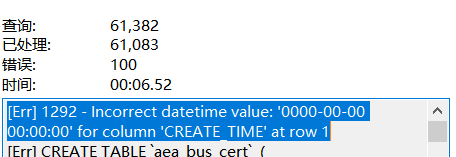# 一、从计算机到Python

## 1.计算机与程序思维

CPU用于进行计算，硬盘用于存储数据和文件，内存（包括缓存）用于连接CPU和硬盘，作为两者的缓冲，可以加快读取和处理速率。

• 学习基本语法
• 读别人的代码
• 修改别人的代码
• 开始写自己的小程序
• 参与开源项目

• 问题表述 有什么，要实现什么。
• 设计计划 如何实现，需要什么。
• 编码与调试 编码与错误验证。
• 评价 评价是否合理。
• 改进（重构） 优化或重新编写。

• 词汇 变量Variables和保留词汇Reserved words。 Python中的保留词汇包括FALSE、class、is、finally、return、None、if、for、lambda、continue、True、def、from、while、nonlocal、and、del、global、with、not、elif、yield、try、as、or、else、assert、import、pass、break、in、raise、except等。
• 句子结构
• 语法Syntax
• 语义Semantics
• 程序结构 为某个目的构造程序。

• input数据输入 从键盘、文件或其他设备获取数据。
• output输出 在屏幕上显示数据或将数据发送到文件或其他设备。
• math计算 执行基本的数学运算，如加法和乘法。
• conditional execution条件执行 检查某些情况并执行适当的代码。
• repetition重复 重复地做一些动作，通常有一些变化。

• Integer整型
• 3、100等整数。
• Float浮点型 带小数点的数字，如3.14159，或某些指数，如1.0e8或10000.0。
• Boolean布尔型 其值为True或False。
• String字符串 文本字符序列。

## 2.Python的特点和应用

Python是一门计算机程序语言，可以理解为人对计算机的指令。

Python之所以这么火，与它的特点有关：

• 简洁美观
• 广泛的第三方库支持 很多人基于Python开发出现成的功能，别人可以直接使用，并且体系逐渐完善，使用起来越来越方便。
• 支持大多数现代云平台
• 最新机器学习框架

• 易学
• 简单与可读性
• 包支持
• 更快
• 处理大数据

## 3.工具选择和安装

Python存在Python2和Python3两个版本，现在Python2已经停止更新，Python3已逐渐成为主流。 Python最常用的IDE（集成开发环境，lntegrated Development Environment）是PyCharm，但是只有专业版（付费）才支持科学计算和数据分析，社区版（免费）不支持科学计算，因此进行数据分析和科学计算还有其他选择，这里选择专业的科学分析工具集Anaconda，并且选择Python3版本。

• IDE 集成开发环境，即lntegrated Development Environment，如PyCharm。 IDE不仅可以编写代码，还可以运行代码，同时还提供代码高亮、调试、代码上传、版本控制等复杂功能，更适合开发大型工程。

• REPL 全称`Read-Evalulate-Print Loop`，即读取-求值-输出循环，也就是交互式的编程环境，如Jupyter Notebook。 其特点如下： （1）优点

• 笔记本形式

• 良好的实验环境

• 方便生成报告

• 免费

（2）缺点

• 不便于版本控制

• 文件组织形式凌乱

# 二、Python的简单使用

## 1.print函数的使用

``print("Hello World! 你好,世界! Hola mundo!")``

``Hello World! 你好,世界! Hola mundo!``

``````print("Hello World!")
print("Hello Again")
print("This is the 3rd line, \n","and this is also the 3rd line.")
print("This is the 3rd line, \
and this is also the 3rd line.")``````

``````Hello World!
Hello Again
This is the 3rd line,
and this is also the 3rd line.
This is the 3rd line, and this is also the 3rd line.``````

## 2.notebook中进行计算

notebook最主要的功能就是进行计算和数据分析。 输入：

``4+5``

``9``

``````print(4-5)
print(4*5)``````

``````-1
20``````

``print(8 + )``

``````File "<ipython-input-6-74de9a467743>", line 1
print(8 + )
^
SyntaxError: invalid syntax``````

``print('8+')``

``8+``

``110/12.97``

``8.481110254433307``

``````111*222
333*444``````

``147852``

``````print(111*222)
print(333*444)``````

``````24642
147852``````

``display(111*222, 333*444)``

``````24642

147852``````

## 3.Python基本语法规范

`=`是赋值运算符，如下：

``number_of_cars = 34``

Python中，有时候空格是有意义的，一般代码用4个空格或者制表符Tab进行缩进、控制代码结构。 如下：

``````x = 8
if x < 7:
print(x)``````

``````File "<ipython-input-13-29d07b2c12b7>", line 4
print(x)
^
IndentationError: expected an indented block``````

``````x = 8
if x < 7:
print(x)``````

``````x=8
if x < 7:
print("x is smaller than 7")
print("x is bigger than 7")``````

``````speed_of_liuxiang=110/12.97
distance= 1000
time = distance/speed_of_liuxiang
print(time)
print(time/60)``````

``````117.9090909090909
1.965151515151515``````

# 三、Python基本数值数据类型

Python中有4种内置数值数据类型：

• 整型int
• 浮点型float
• 布尔型bool
• 字符串str

## 1.整型

``123``

``123``

``````File "<ipython-input-21-65e6e002a62d>", line 1
05
^
SyntaxError: leading zeros in decimal integer literals are not permitted; use an 0o prefix for octal integers``````

``123,456,789``

``(123, 456, 789)``

``````a = 5
a += 2
display(1 + 2, a, a-3,9//5,9/5,9%5,2+3*4)``````

``````3

7

4

1

1.8

4

14``````

``````a = 10**100
display(a, a*a)``````

``````10000000000000000000000000000000000000000000000000000000000000000000000000000000000000000000000000000

100000000000000000000000000000000000000000000000000000000000000000000000000000000000000000000000000000000000000000000000000000000000000000000000000000000000000000000000000000000000000000000000000000000``````

``display(10,0b10,0o10,0x10)``

``````10

2

8

16``````

## 2.浮点型

``````a = 98.5
type(a)``````

``float``

Python提供了很多有用的数学函数，包括在math库中。 要使用它们，必须先通过命令`import math`导入。

``````import math

display(math.pi, math.e, math.floor(98.6), math.ceil(98.6), math.pow(2, 3), 2**3, math.sqrt(25))``````

``````3.141592653589793

2.718281828459045

98

99

8.0

8

5.0``````

## 3.布尔型

``display(type(1 < 2), (2 < 1) or (1 < 2), not (2 < 1), (2 < 1) and (1 < 2), (2 == 1), (2 != 1))``

``````bool

True

True

False

False

True``````

## 4.字符串

``````s = 'this is a string'
display(type(s), "this is also a string", "I'm a string")``````

``````str

'this is also a string'

"I'm a string"``````

``````s = "He said: \"I'm a string\""
print(s)``````

``He said: "I'm a string"``

``````long_s = "You can put a long string that \ntakes up multiple lines here"
print(long_s)``````

``````You can put a long string that
takes up multiple lines here``````

``````s = '''
This is the first line
This is the second line
'''
print(s)``````

``````This is the first line
This is the second line``````

## 5.类型转换

``````a = str(98.6)
display(a,type(a))``````

``````'98.6'

str``````

``display(str(True), float('98.6'), int('-123'))``

``````'True'

98.6

-123``````

``display(1 + 2.0, True + 3)``

``````3.0

4``````

``3.88+"28"``

``````---------------------------------------------------------------------------
TypeError                                 Traceback (most recent call last)
<ipython-input-45-7c94633b599a> in <module>
----> 1 3.88+"28"

TypeError: unsupported operand type(s) for +: 'float' and 'str'``````

``````print(int(3.88) + int("28"))
print(int(-2.95) + int("28"))
print(float(3) + float("28"))
print(str(3.88) + str(28))``````

``````31
26
31.0
3.8828``````

# 四、字符串操作

## 1.结合与重复

``````template = "My name is"
name = "Corley"
greeting = template + " " + name + "."
print(greeting)``````

``My name is Corley.``

``````laugh = 5 * "Ha "
print(laugh)``````

``Ha Ha Ha Ha Ha ``

## 2.提取与切片

``````letters = "abcdefghijklmnopqrstuvwxyz"
letters``````

``'a'``

``````letters = "hello world"
letters``````

``````---------------------------------------------------------------------------
IndexError                                Traceback (most recent call last)
<ipython-input-51-17d500830d0d> in <module>
1 letters = "hello world"
----> 2 letters

IndexError: string index out of range``````

``letters[-2]``

``'l'``

## 3.获取长度

``len(letters)``

``11``

## 4.分割和合并

``````lan = "python ruby c c++ swift"
lan.split()``````

``['python', 'ruby', 'c', 'c++', 'swift']``

``````todos = "download python, install, download ide, learn"
todos.split(', ')``````

``['download python', 'install', 'download ide', 'learn']``

``','.join(['download python', 'install', 'download ide', 'learn'])``

``'download python,install,download ide,learn'``

## 5.替换

``````s = 'I like C. I like C++. She likes Python'
s.replace('like', 'hate')``````

``'I hate C. I hate C++. She hates Python'``

``s.replace('like', 'hate', 2)``

``'I hate C. I hate C++. She likes Python'``

## 6.布局

``````align = 'Learn how to align'
display(align.center(30),align.ljust(30),align.rjust(30))``````

``````'      Learn how to align      '

'Learn how to align            '

'            Learn how to align'``````

`strip()`方法用于去掉字符串前后的空格，如下：

``````ralign = align.rjust(30)
ralign.strip()``````

``'Learn how to align'``

## 7.其他操作

``?py_desc.title``

``````Signature: py_desc.title()
Docstring:
Return a version of the string where each word is titlecased.

cased characters have lower case.
Type:      builtin_function_or_method``````

# 五、输入输出和字符串格式化

``````print('%s %s' % ('one', 'two'))
print('{} {}'.format('one', 'two'))
print('%d %d' % (1, 2))
print('{} {}'.format(1, 2))``````

``````one two
one two
1 2
1 2``````

``print('{1} {0}'.format('one', 'two'))``

``two one``

`format()`方法中还可以给参数传递名称，如下：

``print('{action}---{name}'.format(action='run',name='corley'))``

``run---corley``

Python3.6及之后的版本还提供了格式化字符串字面量的用法，即`f-strings`，格式化字符串更加简便。 如下：

``````a = 5
b = 10
print(f'Five plus ten is {a + b} and not {2 * (a + b)}.')``````

``Five plus ten is 15 and not 30.``

``````name = "Corley"
question = "hello"
print(f"Hello, {name}! How's it {question}?")``````

``Hello, Corley! How's it hello?``blmius
2年前
MySQL:[Err] 1292 - Incorrect datetime value: ‘0000-00-00 00:00:00‘ for column ‘CREATE_TIME‘ at row 1Jacquelyn38
2年前
2020年前端实用代码段，为你的工作保驾护航helloworld_34035044
1年前

​在我们用皕杰报表工具设计填报报表时，如何在新增行里自动增加id呢？能新增整数排序id吗？目前可以在新增行里自动增加id，但只能用uuid函数增加UUID编码，不能新增整数排序id。uuid函数说明：获取一个UUID，可以在填报表中用来创建数据ID语法：uuid()或uuid(sep)参数说明：sep布尔值，生成的uuid中是否包含分隔符''，缺省为Stella981
1年前
Python3：sqlalchemy对mysql数据库操作，非sql语句
Python3：sqlalchemy对mysql数据库操作，非sql语句python3authorlizmdatetime2018020110:00:00coding:utf8'''Wesley13
1年前
4castStella981
1年前
Python之time模块的时间戳、时间字符串格式化与转换
Python处理时间和时间戳的内置模块就有time，和datetime两个，本文先说time模块。关于时间戳的几个概念时间戳，根据1970年1月1日00:00:00开始按秒计算的偏移量。时间元组（struct_time），包含9个元素。 time.struct_time(tm_yWesley13
1年前
mysql设置时区
mysql设置时区mysql\_query("SETtime\_zone'8:00'")ordie('时区设置失败，请联系管理员！');中国在东8区所以加8方法二：selectcount(user\_id)asdevice,CONVERT\_TZ(FROM\_UNIXTIME(reg\_time),'08:00','0Wesley13
1年前
00：Java简单了解Stella981
1年前Wesley13
1年前
MySQL部分从库上面因为大量的临时表tmp_table造成慢查询CuterCorley
Lv1

108

1

6